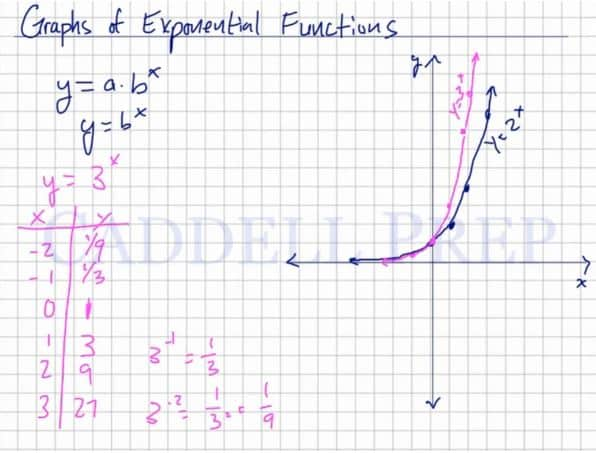In this video, we are going to look at graphs of exponential functions.

• [nonmember]
[/nonmember]

For example:
The graph of$y=2^x$
grows steeper in a positive direction as x values increase after plotting points as shown in the video.
The graph of$y=-(2)^x$
grows steeper in a negative direction as x values increase after plotting points, and is a reflection over the x-axis of the first graph.
The graph of$y=(\frac{1}{2})^x$
grows steeper at a slower rate as x values increase after plotting points, and is a reflection over the y-axis of$y=2^x$## Video-Lesson Transcript

Let’s go over graphs of exponential functions.

First of all, what is an exponential function?

Exponential function has a form of$y = a \times b^x$

Something that should stand out is that$x$ is any exponent. And$a$ and$b$ are constant.

Let’s first look at functions that$a = 1$.

So, we’ll solve for$b$ and$x$.

For demonstration purposes, let’s have$y = 2^x$

Let’s look at some specific ones.

When$x = -2$ then$y = \dfrac{1}{4}$,$x = -1$ then$y = \dfrac{1}{2}$,$x = 0$ then$y = 1$,$x = 1$ then$y = 2$,$x = 2$ then$y = 4$,
and$x = 3$ then$y = 8$.

The thing I want to point out is when$x = 0$ then$y = 1$.

When we graph the positive exponent, it will not be a straight line. It increases at a much higher rate. It even curves up drastically.

When we graph the negative exponents, it will curve but will not touch the$x$-axis.

Because even if the exponent is negative, it just changes to a fraction and not a negative number.

So this is the basic graph of an exponential fraction.

This is$y = 2^x$.

Let’s look at the graph of$y = 3^x$.

Let us have the same points here.

Let’s start with$x = 0$ then$y = 1$.

When$x = -2$ then$y = \dfrac{1}{9}$,$x = -1$ then$y = \dfrac{1}{3}$,$x = 0$ then$y = 1$,$x = 1$ then$y = 3$,$x = 2$ then$y = 9$,
and$x = 3$ then$y = 27$.

This one is very steep on the positive exponents and very low on the negative exponents.

But still won’t touch the$x$-axis.

Let’s graph$y = -(2)^x$.

Let me change the$x$ values a little bit.

Again let’s start with$x = 0$.$y = -1$

When$x = 1$ then$y = -2$,
when$x = 2$ then$y = -4$,
and when$x = 3$ then$y = -8$.

If you look at it, it’s a mirror image of our first example.

When$x = -1$ then$y = - \dfrac{1}{2}$,
when$x = -2$ then$y = - \dfrac{1}{4}$,
and$x = -3$ then$y = - \dfrac{1}{8}$.

It will be very close to the$x$-axis.

The negative will be reflected in the$x$-axis.

Let’s look at another one.

Let’s have$y = (\dfrac{1}{2})^x$

So let’s start with$x = 0$ again,$y = 1$ because any number raised to the power of$0$ is$1$.

When$x = 1$ then$y = \dfrac{1}{2}$,
when$x = 2$ then$y = \dfrac{1}{4}$,
and when$x = 3$ then$y = \dfrac{1}{8}$.

Let’s go backward.

When$x = -1$ then$y = 2$,
when$x = -2$ then$y = 4$,
and when$x = -3$ then$y = 8$.

Now let’s graph this.

Compared to the first example, this graph is reflected in the$y$-axis.

We have a constant that’s greater than$1$ raised to an exponent.
It will constantly raise on one side if the exponent is greater than$0$. Since you’re multiplying it by itself over and over again.
If you have a base that’s greater than$1$, you will have a line that will grow rapidly.
But if you have a base that’s less than$1$ but still positive, it’s going to decrease or decay.